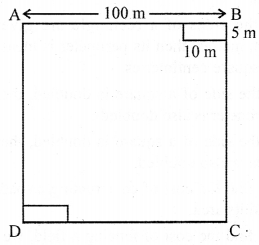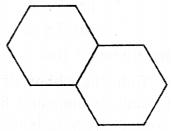## ML Aggarwal Class 6 Solutions for ICSE Maths Chapter 14 Mensuration Objective Type Questions

Mental Maths
Question 1.
Fill in the blanks:
(i) The perimeter of a closed plane figure is the length of its ……….
(ii) The unit of measurement of perimeter is same as that of ……….
(iii) If the side of a rhombus is 7 cm then its perimeter is ……….
(iv) The area of a closed plane figure is measured in ……….
Solution:
(i) The perimeter of a closed plane figure is the length of its boundary.
(ii) The unit of measurement of perimeter is same as that of length.
(iii) If the side of a rhombus is 7 cm then its perimeter is 4 × 7 cm = 28 cm.
(iv) The area of a closed plane figure is measured in sq. units.

Question 2.
State whether the following statements are true (T) or false (F):
(i) Centimetre is the unit of area.
(ii) The sum of lengths of a polygon is called its area.
(iii) If the sides of a rectangle are given in centimetres, then its perimeter is measured in square centimetres.
(iv) If the side of a square is doubled, then its perimeter is also doubled.
(v) If the side of a square is doubled, then its area is also doubled.
(vi) To find the cost of constructing a road, we find its area.
(vii) To find the cost of fencing a field, we find its perimeter.
Solution:
(i) Centimetre is the unit of area. False
(ii) The sum of lengths of a polygon is called its area. False
(iii) If the sides of a rectangle are given in centimetres,
then its perimeter is measured in square centimetres. False
(iv) If the side of a square is doubled, then its perimeter is also doubled. True
(v) If the side of a square is doubled, then its area is also doubled. False
(vi) To find the cost of constructing a road, we find its area. True
(vii) To find the cost of fencing a field, we find its perimeter. True

Multiple Choice Questions
Choose the correct answer from, the given four options (3 to 15):
Question 3.
If the perimeter of a square is 50 cm, then its side is
(a) 200 cm
(b) 150 cm
(c) 25 cm
(d) 12.5 cm
Solution:
Perimeter of a square = 50 cm
⇒ 4 × length of a side = 50 cm
⇒ length of a side = $$\frac{50}{4}$$ cm = 12.5 cm (d)

Question 4.
The area of the rectangle with length 25 cm and breadth 12 cm is
(a) 300 sq. m
(b) 74 cm
(c) 300 sq. cm
(d) 74 sq. cm
Solution:
Length = 25 cm
= 25 cm × 12 cm
= 300 sq. cm (c)

Question 5.
If the perimeter of a square is 36 cm, then its area is
(a) 6 sq. cm
(b) 9 sq. cm
(c) 18 sq. cm
(d) 81 sq. cm
Solution:
Perimetr of a square = 36 cm
⇒ 4 × length of a side = 36
⇒ length of a side = $$\frac{36}{4}$$ = 9 cm
Area of a square = (Length of a side)= (9 cm)2 = 81 cm2 (d)

Question 6.
If the area of rectangular plot is 180 sq. m and its length is 15 m, then its breadth is
(a) 12 m
(b) 12 cm
(c) 60 m
(d) 9 m
Solution:
Length = 15 m
Area of a rectangle = 180 sq. m
Length × Breadth = 180 sq. m
Breadth = $$\frac{180}{15}$$ = 12 m (a)

Question 7.
If the length and the breadth of a rectangle are doubled, then its perimeter
(a) remains the same
(b) doubles
(c) becomes four times
(d) becomes half
Solution:
Let the length of rectangle = l
Let the breadth of rectangle = b
Perimeter = 2(l + b)
If length and breadth are doubles then
Length = 2l
Perimeter = 2(2l + 2b) = 2 × 2(l +b)
Hence perimeter becomes doubles. (b)

Question 8.
If the length and the breadth of a rectangular are doubled then its area
(a) remains same
(b) becomes half
(c) doubles
(d) becomes four times.
Solution:
If length = x
Then area of rectangle = x × y = xy
And If length and breadth are doubled
i. e. length = 2x and breadth = 2y
Then area of rectangle becomes = 2x × 2y = 4 xy
Hence, It shows that the area of rectangle becomes four times. (d)

Question 9.
If the sides of a square are halved, then its area
(a) remains same
(b) becomes half
(c) becomes one-fourth
(d) doubles
Solution:
Let us assume side of a square = x cm
∴ Area = (x)2 = x2 cm2
and if we half the side
∴ New side of a square = $$\frac{x}{2}$$ cm
∴ Area = $$\frac{x^{2}}{4}$$ cm2
Hence, It shows if side of square are halved,
then its area become one-fourth. (c)

Question 10.
A square-shaped park ABCD of side 100 m has two equal flower beds of size 10 m x 5 m as show in the given figure. The perimeter of the remaining park is(a) 340 m
(b) 370 m
(c) 400 m
(d) 430 m
Solution:
Perimeter of square = 4 × Side = 4 × 100 m = 400 m (c)

Question 11.
In the given figure, a square of side 1 cm is joined to a square of side 3 cm. The perimeter of the new figure is(a) 13 cm
(b) 14 cm
(c) 15 cm
(d) 16 cm
Solution:
The given figure isPerimeter = AB + BC + CD + DE + EF + FG + GA
As we know all the sides of square are equal
∴ AB = BC = CD = DA = 3 cm
Also, ED = DG = GF = FE = 1 cm
But in perimeter we need AG
AG = AD – GD = 3 cm – 1 cm = 2 cm
Hence perimeter = (3 + 3 + 3 + 1 + 1 + 1 + 2) cm = 14 cm (b)

Question 12.
Two regular hexagons of perimeter 30 cm each are joined as shown in the given figure. The perimeter of the new figure is(a) 65 cm
(b) 60 cm
(c) 55 cm
(d) 50 cm
Solution:
As per given figure,Perimeter = 30 cm
∴ Side = $$\frac{30}{6}$$ = 5 cm
Hence, remaining perimeter
= AB + BC + CD + DE + EF + FG + GH + HI + IJ + JA
= (5 + 5 + 5 + 5 + 5 + 5 + 5 + 5 + 5 + 5) cm
= 50 cm (d)

Question 13.
If the area of a square is numerically equal to its perimeter, then the length of each side is
(a) 1 unit
(b) 2 units
(c) 3 units
(d) 4 units
Solution:
Let the side of the square be s
∴ Area = (side)2 = s2
And perimeter = 4 × length of the side = 4 s
But, we have, area = perimeter
∴ s2 = 4s ⇒ s = 4
∴ The length of each side is 4 units (d)

Question 14.
If a ribbon of length 10 m is stitched around a rectangular table cloth making 2 rounds along its boundary, then the perimeter of the table cloth is
(a) 20 m
(b) 10 m
(c) 5 m
(d) 2.5 m
Solution:
Length of the Ribbon = 10m
As per the question,
the ribbon of length is stitched around a rectangular table
cloth-making 2 rounds along with its bounding
∴ Perimeter of the table clothQuestion 15.
A picture is 60 cm wide and 1.8 m long. The ratio of its width to its perimeter in lowest form is
(a) 1 : 2
(b) 1 : 3
(c) 1:6
(d) 1 : 8
Solution:
Width of picture = 60 cm
Length = 1.8 m = 180 cm
Perimeter = 2(l + b) = 2(60 + 180) = 2(240) = 480 cm
Ratio = $$\frac{60}{480}=\frac{1}{8}=1 : 8$$ (d)

Higher Order Thinking Skills (Hots)
Question 1.
How many envelopes of size 25 cm x 15 cm can be made from a rectangular sheet of size 4 m x 1.2 m?
Solution:
Size of envelope = 25 cm × 15 cm
Area of envelope = 25 × 15 cm2 = 375 cm2
Size of rectangular sheet = 4 m × 1.2 m
Area of rectangular sheet = 400 cm × 120 cm = 48000 cm2Question 2.
The perimeter of a rectangle is 36 cm. What will be length and breadth (in natural number) of that rectangle whose area is
(i) maximum?
(ii) minimum?
Solution:
(i) When area is maximum Then, l = 9 cm, b = 9 cm
(ii) When area is minimum Then, l = 17 cm b = 1 cm